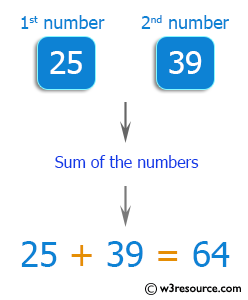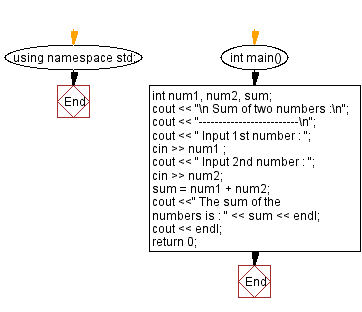﻿ C++ Exercises: Add two numbers accept through keyboard - w3resource

# C++ Exercises: Add two numbers accept through keyboard

## C++ Basic: Exercise-12 with Solution

Write a C++ program to add two numbers and accept them from the keyboard.

Visual Presentation:Sample Solution:

C++ Code :

``````#include <iostream> // Including the input-output stream header file

using namespace std; // Using the standard namespace

int main() // Start of the main function
{
int num1, num2, sum; // Declaring integer variables num1, num2, and sum

cout << "\n Sum of two numbers :\n"; // Outputting a message indicating sum of two numbers
cout << "-------------------------\n"; // Outputting a separator line

cout << " Input 1st number : "; // Prompting the user to input the first number
cin >> num1 ; // Taking input for the first number from the user

cout << " Input 2nd number : "; // Prompting the user to input the second number
cin >> num2; // Taking input for the second number from the user

sum = num1 + num2; // Calculating the sum of the two numbers

cout <<" The sum of the numbers is : " << sum << endl; // Displaying the sum of the numbers
cout << endl; // Outputting a blank line for better readability

return 0; // Returning 0 to indicate successful program execution
} // End of the main function

``````

Sample Output:

``` Sum of two numbers :
-------------------------
Input 1st number : 25
Input 2nd number : 39
The sum of the numbers is : 64
```

Flowchart:C++ Code Editor:

What is the difficulty level of this exercise?

﻿